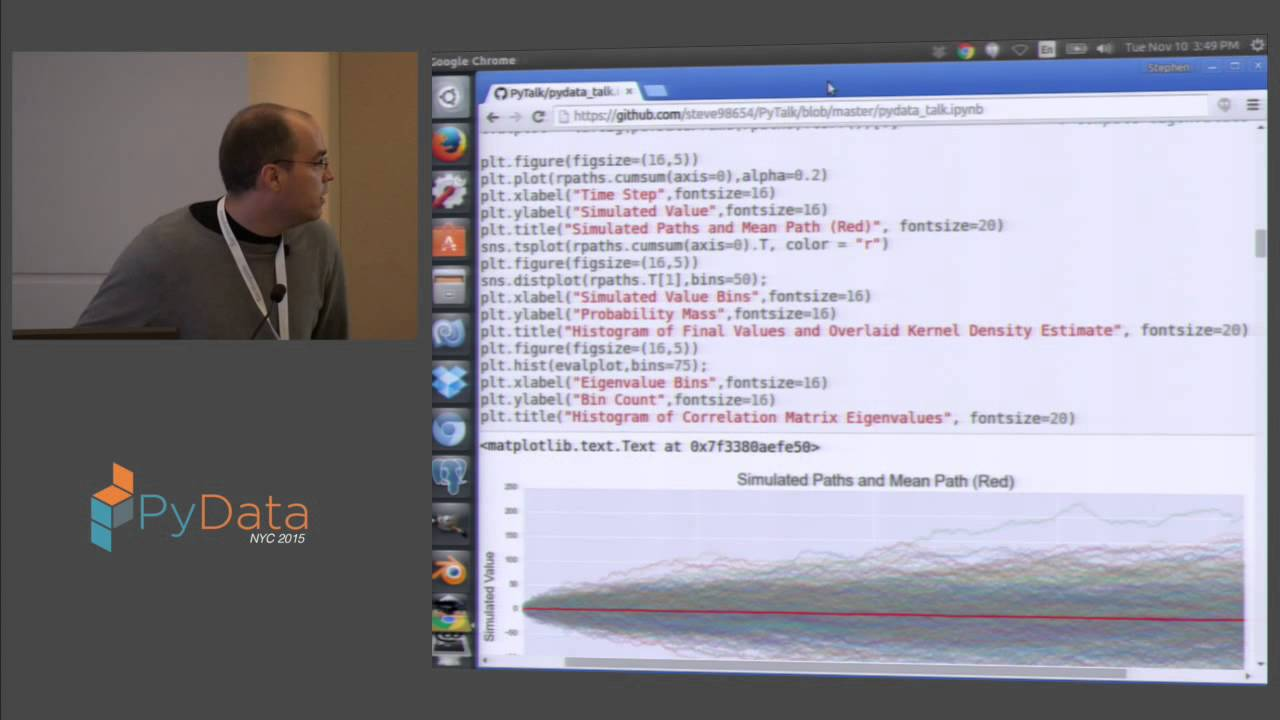# Steve Taylor: Correlation Matrix Filtering and Asset Allocation with Python

PyData NYC 2015
We use tools from Pandas, NumPy, and SciPy to implement a correlation matrix filtering algorithm of Marcenko and Paster. The filtered correlation matrix is then converted to a covariance matrix which is used as an input into Markowitz’s mean/variance asset allocation method. We discuss differences between optimal portfolio weights using both the raw and filtered covariance matricies.

Correlation matrices provide a means of encoding pairwise dependency relationships between time series and are a key input parameter into a wide variety of financial models. However, they are prone to estimation error due to outliers and poor estimates may have significant impact on subsequent model results. We examine one technique for reducing this error, and consider how the raw and filtered covariance matrices alter the optimal portfolio in Markowitz’s Modern Portfolio Theory. Specifically, we first specify, download, and wrangle, end of day price time series for the majority of exchange traded US equity securities from Yahoo! Finance. Next, we review a result in random matrix theory of Marcenko and Pastur about the distribution of eigenvalues of correlation matrices constructed from random time series as well as financial applications of their result by Bouchaud and Potters. These ideas are then applied to filtering a correlation matrix constructed from the end of day stock prices. Finally, we compute Markowitz’s optimal portfolio using both the raw and filtered correlation/covariance matrices and discuss asset allocation differences between these two models.

Slides available here:

Nguồn: https://benjaminjcohen.com/

Xem thêm bài viết khác: https://benjaminjcohen.com/cong-nghe/